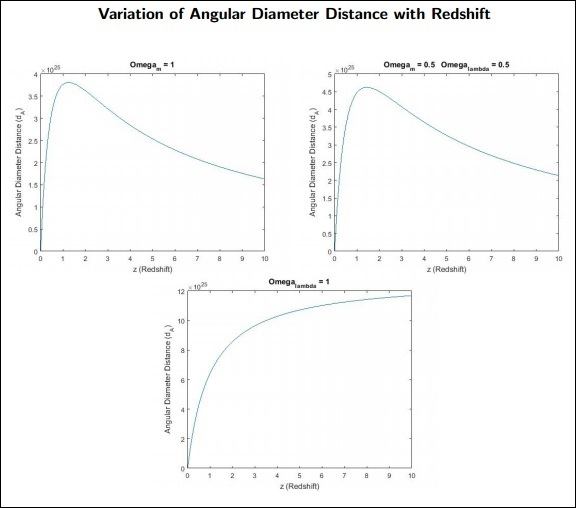# Cosmology - Angular Diameter Distance

In this chapter, we will understand what the Angular Diameter Distance is and how it helps in Cosmology.

For the present universe −

• $\Omega_{m,0} \: = \: 0.3$

• $\Omega_{\wedge,0} \: = \: 0.69$

• $\Omega_{rad,0} \: = \: 0.01$

• $\Omega_{k,0} \: = \: 0$

We’ve studied two types of distances till now −

• Proper distance (lp) − The distance that photons travel from the source to us, i.e., The Instantaneous distance.

• Comoving distance (lc) − Distance between objects in a space which doesn’t expand, i.e., distance in a comoving frame of reference.

## Distance as a Function of Redshift

Consider a galaxy which radiates a photon at time t1 which is detected by the observer at t0. We can write the proper distance to the galaxy as −

$$l_p = \int_{t_1}^{t_0} cdt$$

Let the galaxy’s redshift be z,

$$\Rightarrow \frac{\mathrm{d} z}{\mathrm{d} t} = -\frac{1}{a^2}\frac{\mathrm{d} a}{\mathrm{d} t}$$

$$\Rightarrow \frac{\mathrm{d} z}{\mathrm{d} t} = -\frac{\frac{\mathrm{d} a}{\mathrm{d} t}}{a}\frac{1}{a}$$

$$\therefore \frac{\mathrm{d} z}{\mathrm{d} t} = -\frac{H(z)}{a}$$

Now, comoving distance of the galaxy at any time t will be −

$$l_c = \frac{l_p}{a(t)}$$

$$l_c = \int_{t_1}^{t_0} \frac{cdt}{a(t)}$$

In terms of z,

$$l_c = \int_{t_0}^{t_1} \frac{cdz}{H(z)}$$

There are two ways to find distances, which are as follows −

### Flux-Luminosity Relationship

$$F = \frac{L}{4\pi d^2}$$

where d is the distance at the source.

### The Angular Diameter Distance of a Source

If we know a source’s size, its angular width will tell us its distance from the observer.

$$\theta = \frac{D}{l}$$

where l is the angular diameter distance of the source.

• θ is the angular size of the source.

• D is the size of the source.

Consider a galaxy of size D and angular size .

We know that,

$$d\theta = \frac{D}{d_A}$$

$$\therefore D^2 = a(t)^2(r^2 d\theta^2) \quad \because dr^2 = 0; \: d\phi ^2 \approx 0$$

$$\Rightarrow D = a(t)rd\theta$$

Changing r to rc, the comoving distance of the galaxy, we have −

$$d\theta = \frac{D}{r_ca(t)}$$

Here, if we choose t = t0, we end up measuring the present distance to the galaxy. But D is measured at the time of emission of the photon. Therefore, by using t = t0, we get a larger distance to the galaxy and hence an underestimation of its size. Therefore, we should use the time t1.

$$\therefore d\theta = \frac{D}{r_ca(t_1)}$$

Comparing this with the previous result, we get −

$$d_\wedge = a(t_1)r_c$$

$$r_c = l_c = \frac{d_\wedge}{a(t_1)} = d_\wedge(1+z_1) \quad \because 1+z_1 = \frac{1}{a(t_1)}$$

Therefore,

$$d_\wedge = \frac{c}{1+z_1} \int_{0}^{z_1} \frac{dz}{H(z)}$$

dA is the Angular Diameter Distance for the object.### Points to Remember

• If we know a source’s size, its angular width will tell us its distance from the observer.

• Proper distance is the distance that photons travel from the source to us.

• Comoving distance is the distance between objects in a space which doesn’t expand.